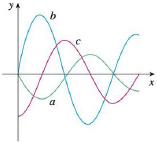Chapter 5, Problem 7RE### Single Variable Calculus: Early Tr...

8th Edition
James Stewart
ISBN: 9781305270343

#### Solutions

Chapter
Section### Single Variable Calculus: Early Tr...

8th Edition
James Stewart
ISBN: 9781305270343
Textbook Problem

# The figure shows the graphs of f, f′, and ∫ 0 x f ( t )   d t . Identify each graph, and explain your choices.To determine

To identify: The graphs of f, f and 0xf(t)dt.

Explanation

Given information:

Show the graphs of f, f and 0xf(t)dt as in Figure 1.

Calculation:

Refer to Figure 1.

Consider the explanation of graph as follows:

• f(t) is the double derivative of 0xf(t)dt

### Still sussing out bartleby?

Check out a sample textbook solution.

See a sample solution

#### The Solution to Your Study Problems

Bartleby provides explanations to thousands of textbook problems written by our experts, many with advanced degrees!

Get Started